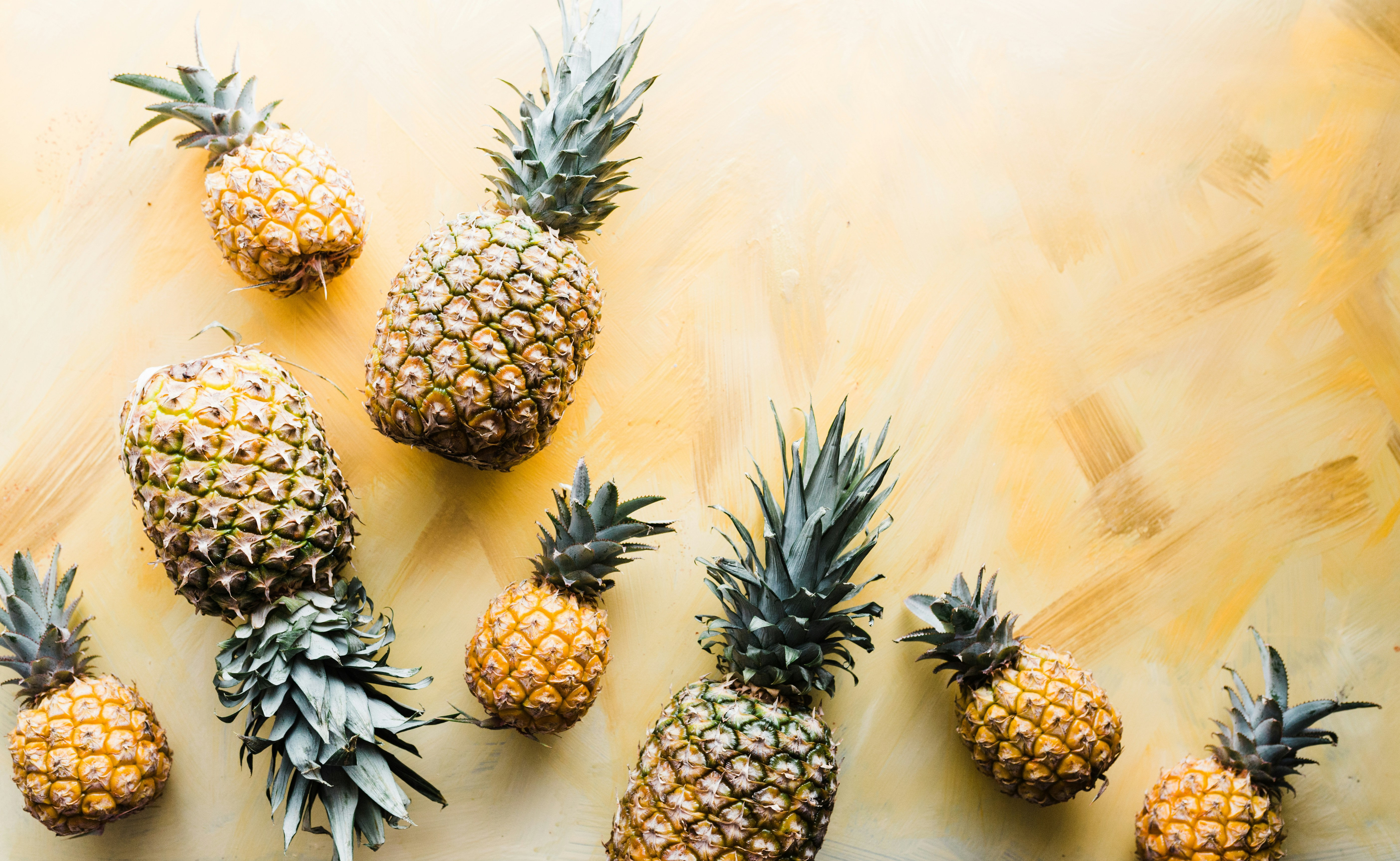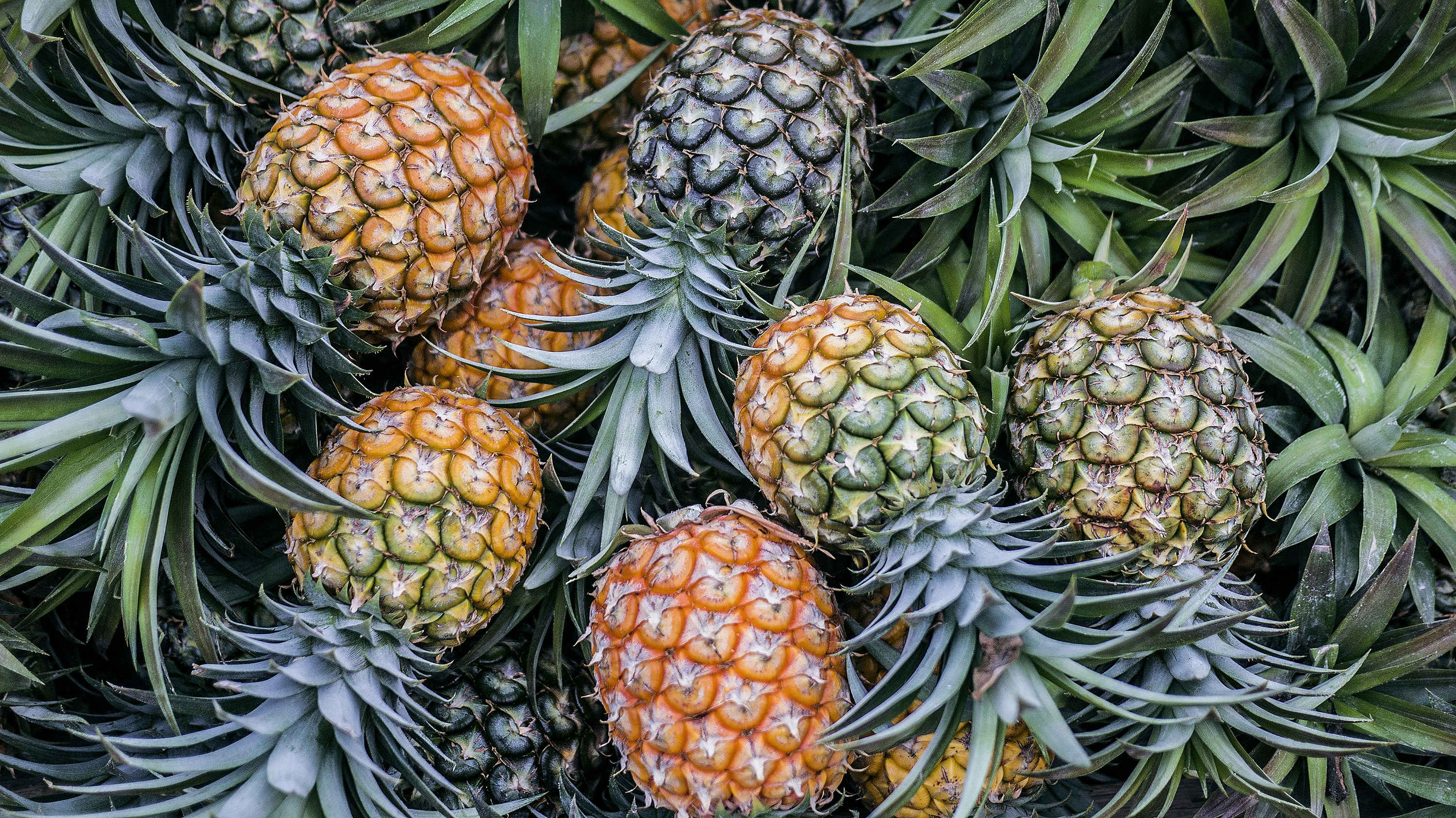# It's a Bird, It's a Plane ... It's a ggforce function

ggforce is a powerful package that extends heavily the ggplot2 API. In this blog post, I won’t go through all its capabilities, I’ll just provide a tiny sample of some cool functions that can be useful for ggplot2 coders. Let’s get started !from Unsplash by Brooke Lark

# theme_no_axes()

`theme_no_axes()` removes the axis text, title, ticks and grid lines for most themed plot, particularly useful in some cases:

``````library(tidyverse)
library(ggforce)

ggplot(mpg, aes(hwy, cty)) +
geom_point() +
theme_no_axes(base.theme = theme_dark()) ````````````ggplot(mpg, aes(hwy, cty)) +
geom_point() +
theme_no_axes(base.theme = ggthemes::theme_economist_white()) ``````# facet_zoom()

`facet_zoom()` zooms on a particular data category within a plot. Below we’ll use the `starwars` data frame and zoom on the x-axis of the female category from the sex variable.

``````ggplot(starwars, aes(mass, height, col = sex)) +
geom_point(na.rm = TRUE) +
facet_zoom(x = sex == "female", zoom.size = 2) +
ggthemes::theme_hc()``````# facet_row()

`facet_row()` is a one-dimensional version of `facet_wrap()`. It aligns all the subplot into one row:

``````library(palmerpenguins)

ggplot(penguins) +
geom_point(aes(bill_length_mm, body_mass_g), col = "white", na.rm = TRUE) +
facet_row(~island) +
theme_no_axes(base.theme = theme_dark()) ```````facet_col` does the same thing except that it arranges sub-plots within one column :

``````ggplot(penguins) +
geom_point(aes(bill_length_mm, body_mass_g), col = "white", na.rm = TRUE) +
facet_col(~island) +
theme_no_axes(base.theme = theme_dark()) ``````From Unsplash by Phoenix Han

# facet_matrix()

Using `facet_matrix()` you can specify a matrix composed of inter-related plots. In the example below, we’ll map `bill_length_mm`, `bill_depth_mm` and `flipper_length_mm` (on the x-axis) with `body_mass_g` (on the y-axis):

``````ggplot(penguins, aes(x = .panel_x, y = .panel_y, col = sex)) +
geom_point(na.rm = TRUE) +
facet_matrix(rows = vars(body_mass_g),
cols = vars(bill_length_mm, bill_depth_mm, flipper_length_mm),
switch = "x")``````You can remove the `x = .panel_x, y = .panel_y` part using the `geom_autopoint()` function:

``````ggplot(penguins, aes(col = sex)) +
geom_autopoint(na.rm = TRUE) +
facet_matrix(rows = vars(body_mass_g),
cols = vars(bill_length_mm, bill_depth_mm, flipper_length_mm),
switch = "x")``````In the same way, you can get an overview of the different interactions between many numerical variables:

``````ggplot(penguins, aes(col = sex)) +
geom_autopoint(na.rm = TRUE) +
facet_matrix(rows = vars(bill_length_mm:body_mass_g),
switch = "x")``````It’s also possible to include interactions between numerical and categorical variables (be sure to drop missing values beforehand):

``````ggplot(penguins %>% drop_na(), aes(col = sex)) +
geom_autopoint() +
facet_matrix(rows = vars(island:body_mass_g),
switch = "x") +
theme(axis.text.x = element_text(angle = 90))``````Further, it’s possible to plot histograms within the diagonal of the matrix using the `geom_autohistogram()` function:

``````ggplot(penguins %>% drop_na(), aes(col = sex, fill = sex)) +
geom_autopoint() +
facet_matrix(rows = vars(island:body_mass_g),
switch = "x", layer.diag = 2) +
theme(axis.text.x = element_text(angle = 90)) +
geom_autohistogram()``````Here the `layer.diag` argument determines which layer will be used within the diagonal. In this case, we’ve specified the value 2 which corresponds to the second layer: `geom_autohistogram()`.

In the same manner, we can use `geom_autodensity()` to plot densities:

``````ggplot(penguins %>% drop_na(), aes(col = sex, fill = sex)) +
geom_autopoint() +
facet_matrix(rows = vars(island:body_mass_g),
switch = "x", layer.diag = 2) +
theme(axis.text.x = element_text(angle = 90)) +
geom_autodensity()``````# geom_mark_ellipse()

First, we render the following plot:

``````ggplot(penguins %>% drop_na(), aes(bill_depth_mm, body_mass_g, col = species)) +
geom_point(na.rm = TRUE)``````Now, let’s say we want to highlight a particular species (e.g. Gentoo), we’ll use the `geom_mark_ellipse()` as follows:

``````ggplot(penguins %>% drop_na(), aes(bill_depth_mm, body_mass_g)) +
geom_point() +
geom_mark_ellipse(aes(filter = species == "Gentoo",
label = "Gentoo",
description = "Gentoo penguins seem quite different from the other species"),
label.fill = "pink",
label.colour = "red",
con.colour = "red",
con.linetype = 2,
expand = unit(2, "mm")) ``````Note that there’re other `geom_mark_*()` functions (e.g. geom_mark_rect) that you can use:

``````ggplot(penguins %>% drop_na(), aes(bill_depth_mm, body_mass_g)) +
geom_point() +
geom_mark_rect(aes(filter = species == "Gentoo",
label = "Gentoo",
description = "Gentoo penguins seem quite different from the other species"),
label.fill = "pink",
label.colour = "red",
con.colour = "red",
con.linetype = 2,
expand = unit(0.01, "mm"),
fill = "pink") ``````## Thanks for reading !##### Dr. Mohamed El Fodil Ihaddaden, Ph.D

My research interests include Performance Management, Efficiency Analysis and Experimental Economics.